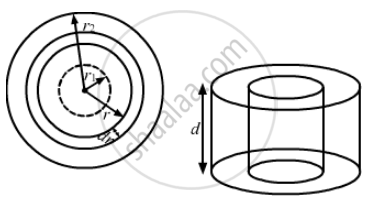Advertisement Remove all ads

# A Hole of Radius R1 is Made Centrally in a Uniform Circular Disc of Thickness D and Radius R2. the Inner Surface (A Cylinder a Length D and Radius - Physics

Sum

A hole of radius r1 is made centrally in a uniform circular disc of thickness d and radius r2. The inner surface (a cylinder a length d and radius r1) is maintained at a temperature θ1 and the outer surface (a cylinder of length d and radius r2) is maintained at a temperature θ2 (θ1 > θ2). The thermal  conductivity of the material of the disc is K. Calculate the heat flowing per unit time through the disc.

Advertisement Remove all ads

#### SolutionLet (d theta)/(dt) be the rate of flow of heat.

Consider an annular ring of radius and thickness dr.

Rate of flow of heat is given by

(d theta)/(dt) = K (2pird)

Rate of flow of heat is constant.

∴ (d theta)/(dt) = i

i = - k ( 2pir.d) (d theta)/(dr)

int_{r_1}^{r_2} dr/r = (2piKd)/l  int_{theta_1}^(theta_2)   d theta

["ln" (r) ]_{r1}^{r2} = (2pikd)/l [ theta_2 - theta 1]

i =(2pikd(theta_2 -theta_1))/("ln"(r_2/r_1)

Concept: Thermal Expansion of Solids
Is there an error in this question or solution?
Advertisement Remove all ads

#### APPEARS IN

HC Verma Class 11, Class 12 Concepts of Physics Vol. 2
Chapter 6 Heat Transfer
Q 20 | Page 99
Advertisement Remove all ads
Advertisement Remove all ads
Share
Notifications

View all notifications

Forgot password?